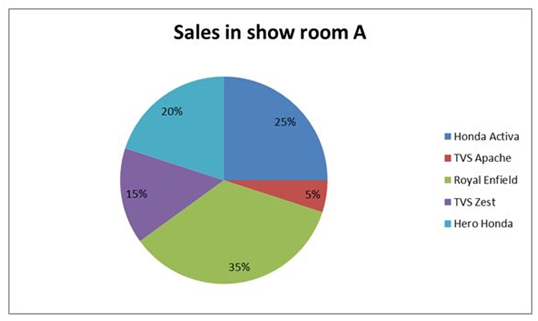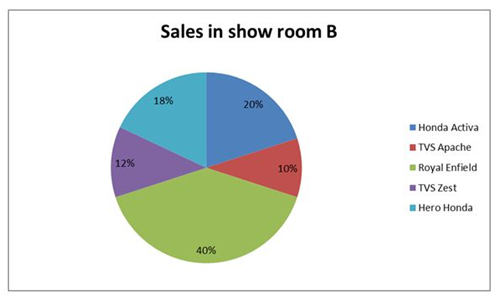# SBI Clerk Mains 2021 Quantitative Aptitude (Day-02)

Dear Aspirants, Our IBPS Guide team is providing new series of Quantitative Aptitude Questions for SBI Clerk Mains 2021 so the aspirants can practice it on a daily basis. These questions are framed by our skilled experts after understanding your needs thoroughly. Aspirants can practice these new series questions daily to familiarize with the exact exam pattern and make your preparation effective.

### Start Quiz

Number series

Directions (1-5): The questions below are based on the given Series-I. The series-I satisfy a certain pattern, follow the same pattern in Series-II and answer the questions given below.

1) I. 8, 4, 6, 15, 52.5

II) 12 …. 78.75. If 78.75 is the nthterm find the value of n

A.6

B.4

C.5

D.7

E.8

2) I) 19, 38, 114, 570, 3990

II) 23 ….. 690. If 690 is the nthterm find the value of n

A.6

B.5

C.7

D.8

E.4

3) I) 15, 32, 68, 144, 304

II) 0.5 ….. 176. If 176 is the nthterm find the value of n

A.9

B.8

C.7

D.6

E.11

4) I) 11, 23, 33, 41, 47

II) 114 …… 154. If 154 is the nthterm find the value of n

A.6

B.3

C.5

D.7

E.4

5) I) 102, 342, 462, 542, 602

II) 7 ….. 447. If 447 is the nthterm find the value of n

A.2

B.3

C.4

D.6

E.5

Pie chart

Directions (06-10): Study the following information carefully and answer the questions given below.

The given pie chart shows the percentage of sales of different brands of two wheelers in showroom A in 2019.The given pie chart shows the percentage of the sales of different brands of two wheelers in showroom B in 2019.6) Ratio of the total sales in showroom A and B is 2:3. If the difference between the sales of Royal Enfield and TVS Apache in showroom A is 300, then what is the difference between the sales of same two brands of two wheelers in showroom B?

A.300

B.420

C.360

D.450

E.480

7) Ratio of the total sales in showroom A to B is 6:5, what is the ratio of the total sales of TVS zest and Honda Activa in showroom A to the total sales of TVS Zest and Hero Honda in showroom B?

A.8:5

B.6:1

C.5:3

D.8:3

E.None of these

8) If the total sales of showroom A and B in the ratio of 2:3, then the total sales of Hero Honda and TVS Apache in showroom A is approximately what percent of the total sales of Honda Activa and TVS Apache in showroom B?

A.39.83%

B.55.55%

C.43.34%

D.48.76%

E.59.87%

9) The total sales in showroom A is 25% less than the total sales in showroom B. What is the difference between the total sales of Royal Enfield and Honda Activa in showroom A and the total sales of TVS Zest and Hero Honda in showroom B, if the total sale in showroom A is 900?

A.120

B.130

C.150

D.180

E.210

10) If the sum of the total sales of Honda Activa in showroom A and B is 3200 and the sales of Hero Honda in showroom A is 520 more than the sales of Hero Honda in showroom B, Then the total sales in showroom A is approximately what percent of the total sales in showroom A and B together?

A.57.14%

B.59.12%

C.43.56%

D.48.82%

E.53.38%

Series – I,

8 is the 1st term

8 * 0.5 = 4

4 * 1.5 = 6

6 * 2.5 = 15

15 * 3.5 = 52.5

Series – II,

12 is the 1st term

12 * 0.5 = 6

6 * 1.5 = 9

9 * 2.5 = 22.5

22.5 * 3.5 = 78.75 = 5th term

Series –I,

19 is the 1st term

19 * 2 = 38

38 * 3 = 114

114 * 5 = 570

570 * 7 = 3990

Series –II

23 is the 1st term

23 * 2 = 46

46 * 3 = 138

138 * 5 = 690 (4th term)

Series –I

15 is the 1st term

15 * 2 + 2 = 32

32 * 2 + 4 = 68

68 * 2 + 8 = 144

144 * 2 + 16 = 304(5th term)

Series – II,

0.5 is the 1st term

0.5 * 2 + 2 = 3

3 * 2 + 4 = 10

10 * 2 + 8 = 28

28 * 2 + 16 = 72

72 * 2 + 32 = 176 = 6th term

Series – I,

11 is the 1st term

11 + 12 = 23

23 + 10 = 33

33 + 8 = 41

41 + 6 = 47 (5th term)

Series – II,

114 is the 1st term

114 + 12 = 126

126 + 10 = 136

136 + 8 = 144

144 + 6 = 150

150 + 4 = 154 (6th term)

Series – I

102 is the 1st term

102 + 240/1 = 342

342 + 240/2 = 462

462 + 240/3 = 542

542 + 240/4 = 602

Series – II,

7 is the 1st term

7 + 240 = 247

247 + 240/2 = 367

367 + 240/3 = 447 (4th term)

Total sales in A = 100/(35 – 5) * 300= (100/30) * 300

= 1000

Number of sales in B = 3/2 * 1000 = 1500

Required difference = (40 – 10)/100 * 1500 = (30/100) * 1500 = 450

Total sales in A = 6x

Total sales in B = 5x

Required ratio = (15 + 25)/100 * 6x: (12 + 18)/100 * 5x

= 24:15

= 8:5

Total sales in A = 900

Total sales in B = 900 * 100/75 = 1200

Number of sales of Royal Enfield and Honda activa in A = (35 + 25)/100 * 900 = (60/100) * 900 = 540

Number of sales of TVS zest and Hero Honda in B = 1200 * (12 + 18)/100 = (30/100) * 1200 = 360

Difference = 540 – 360 = 180

Total sales in A = 900

Total sales in B = 900 * 100/75 = 1200

Number of sales of Royal Enfield and Honda activa in A = (35 + 25)/100 * 900 = (60/100) * 900 = 540

Number of sales of TVS zest and Hero Honda in B = 1200 * (12 + 18)/100 = (30/100) * 1200 = 360

Difference = 540 – 360 = 180

Total sales of A = 100x

Total sales of B = 100y

Number of sales of Honda activa in A = 100x * 25/100 = 25x

Number of sales of Honda activa in B = 100y * 20/100 = 20y

Number of sales of Hero Honda in A = 100x * 20/100 = 20x

Number of sales of Hero Honda in B = 18/100 * 100y = 18y

25x + 20y = 3200—-(1)

20x – 18y = 520—–(2)

From (1) and (2)

x = 80

y = 60

Required percentage = 100 * 80/(80 * 100 + 100 * 60) * 100

= (800/1400) * 100

= 57.14%

5 1 vote
Rating